Importing Data From Excel

Today I want to share a useful tip for importing signals from Excel into a simulation.

In case you were not aware, since R2015b, Simulink has offered the From Spreadsheet block.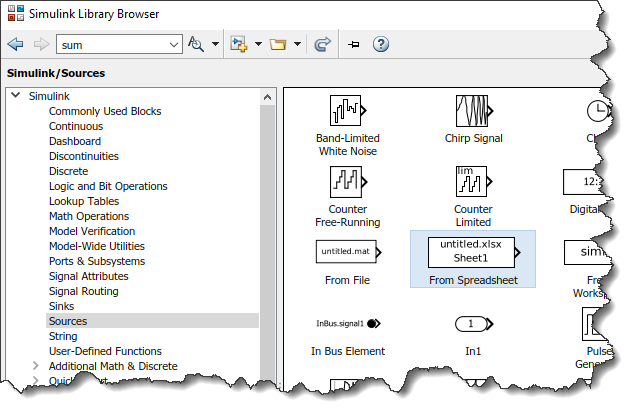Using this block, you can specify an Excel file, sheet name, and range of the data.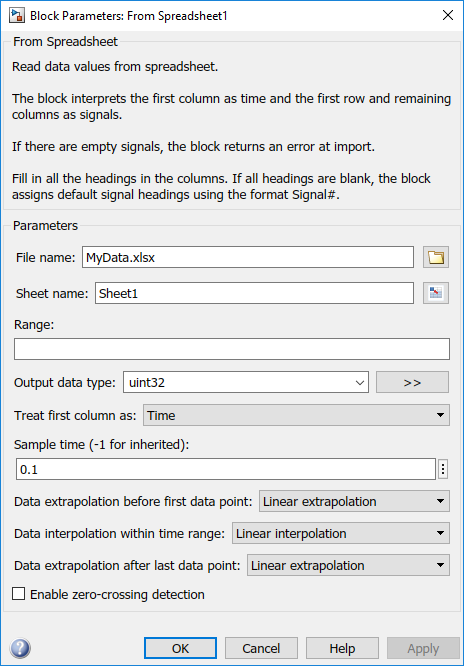Let's take a simple example Excel sheet like the following, where we have points sampled at every 0.1 seconds: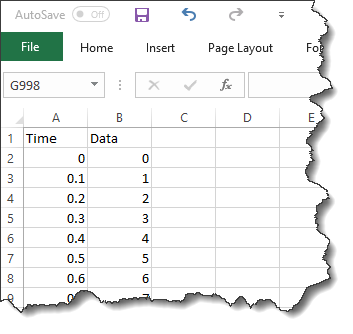If I configure the From Spreadsheet block as in the above image, specifying that the first column is time, I will very likely see either duplicate or skipped points in the simulation.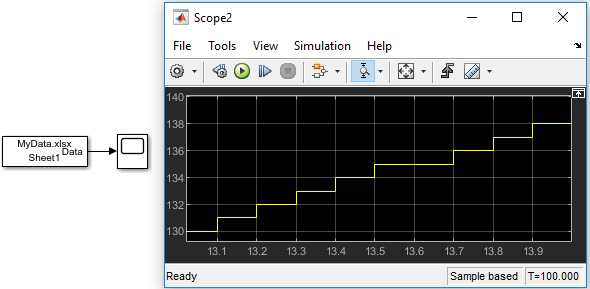Why is that? This is due to the same phenomenon as I explained in this previous post about importing discrete signal data in Simulink

If we compare the "equally spaced" time points from the Excel spreadsheet, you will notice that they are slightly different from the equally spaced time points taken by Simulink: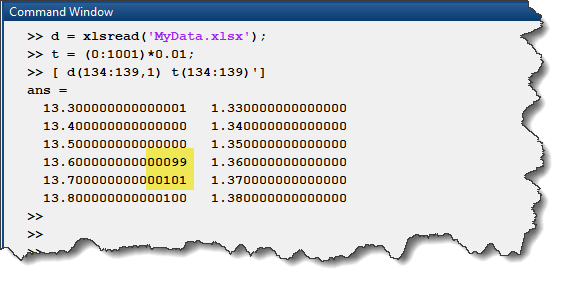In this example, when Simulink takes a point at 13.6sec, it sees that the data contains no point at exactly 13.6sec and holds the previous value from 13.5sec. At 13.7sec, it will output the last point it saw in the data, the one at 13.6000000000099sec.

How can you avoid this situation?

To avoid this confusion between the steps taken by Simulink and the steps specified in the Excel sheet, what I recommend is to configure the From Spreadsheet block to ignore the time column in the Excel sheet.

To do that, in the block dialog, set the Sample Time to the appropriate value, then set the Treat first column as to Data and specify the Range to start at the second column: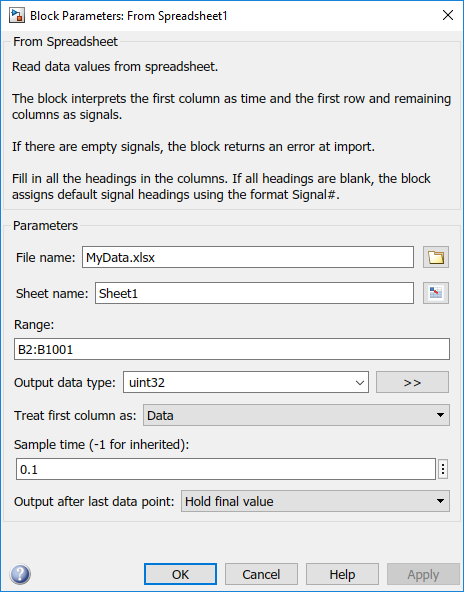That way, the From Spreadsheet block will simply use one row of the Excel sheet at every time step.